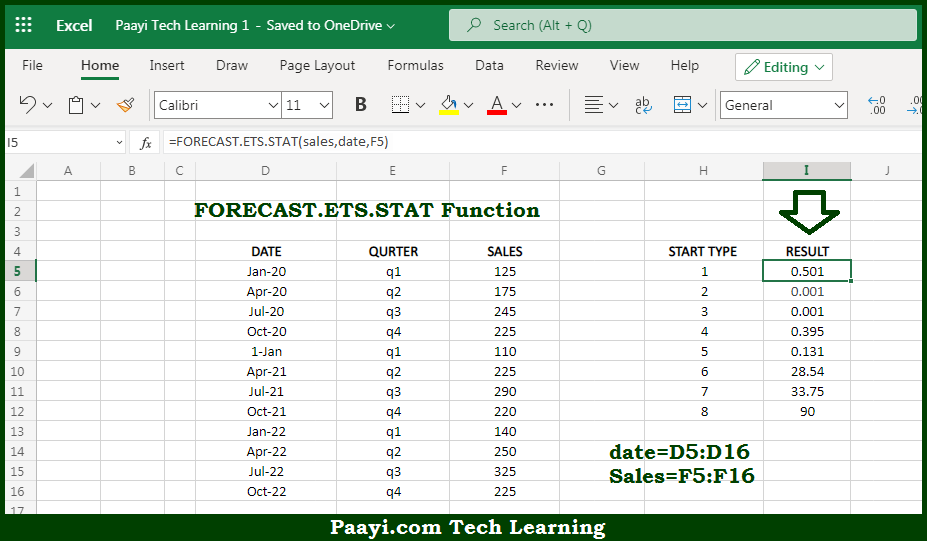# Learn How to Use Microsoft Excel FORECAST.ETS.STAT Function

Written by | 0 Comments | 671 Views

In this article, you will learn how to use Microsoft Excel FORECAST.ETS.STAT function and its prime function in Microsoft Excel. You will also get to know Microsoft Excel FORECAST.ETS.STAT function returns value and syntax with the help of some examples.

Microsoft Excel FORECAST.ETS.STAT Function

The main purpose of the Microsoft Excel FORECAST.ETS.STAT function is to get the statistical value related to forecasting. That implies, with the help of FORECAST.ETS.STAT function you can able to return a particular statistical value related to time series forecasting with the FORECAST.ETS function. The statistic-type argument determines which statistic is returned by FORECAST.ETS.STAT function. So, with the help of FORECAST.ETS.STAT function, you can able to get the statistical value related to forecasting.

Return Value of FORECAST.ETS.STAT Function

The return value will be statistical related to forecasting.

Syntax of FORECAST.ETS.STAT Function

=FORECAST.ETS.STAT(values, timeline, statistic-type, [seasonality] [data-completion], [aggregation])

Where the arguments:

• values: This is the existing or historical values (y values).
• timeline: This is the numeric timeline values (x values).
• statistic-type: This is the statistic to return, a numeric value between 1 and 8
• seasonality: This is the Seasonality calculation, where 0 = no seasonality, 1 = automatic, n = season length in timeline units (optional).
• data-completion: This is the Missing data treatment, where, 0 = treat as zero, 1 = average and default is 1 (optional).
• aggregation: This is the Aggregation behavior. Default is 1 AVERAGE (optional).

## How to Use Microsoft Excel FORECAST.ETS.STAT Function?So we know that, Microsoft Excel FORECAST.ETS.STAT function you can able to get the statistical value related to forecasting. That implies, with the help of FORECAST.ETS.STAT function you can able to return a particular statistical value related to time series forecasting with the FORECAST.ETS function. The statistic-type argument determines which statistic is returned by FORECAST.ETS.STAT function. So, with the help of FORECAST.ETS.STAT function, you can able to get the statistical value related to forecasting.